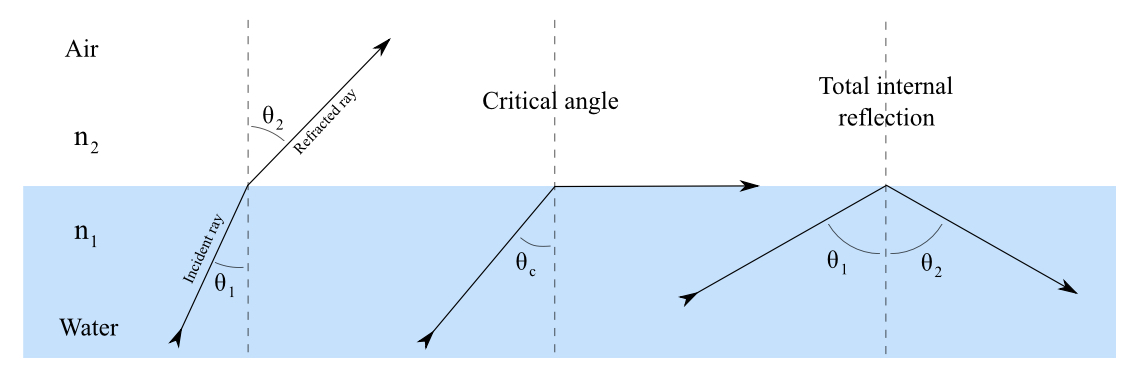The MCAT CARS Strategy Course begins January 28!

MCAT Content / Geometrical Optics / Conditions For Total Internal Reflection

### Conditions for total internal reflection

Topic: Geometrical Optics

Total internal reflection happens when a propagating wave strikes a medium boundary at an angle larger than a particular critical angle.

If the refractive index is lower on the other side of the boundary and the incident angle is greater than the critical angle, the wave cannot pass through and is entirely reflected. The critical angle is the angle of incidence above which the total internal reflectance occurs.Consider a light ray passing from water into air. The light emanating from the interface is bent towards the water. When the incident angle is increased sufficiently, the transmitted angle (in air) reaches 90 degrees. It is at this point no light is transmitted into air—the critical angle θc.One of the most common applications of total internal reflection is in fibre optics. An optical fibre is a thin, transparent fibre, usually made of glass or plastic, for transmitting light. Fibers in bundles are clad by a material that has a lower index of refraction than the core to ensure total internal reflection, even when fibers are in contact with one another. Light can travel in fiber optic over a very long distance without a significant loss.Practice Questions

Eye disease and contact lenses

An experiment with light rays and a prism

MCAT Official Prep (AAMC)

Physics Online Flashcards Question 9

Key Points

• The critical angle is the angle of incidence above which total internal reflection occurs and given as θc = arcsin(n2/n1)

• The critical angle is only defined when n2/n1 is less than 1.

• If light is incident on an optical fiber with an angle of incidence greater than the critical angle then the light will remain trapped inside and travel without loss.

Key Terms

Index of refraction: for a material, the ratio of the speed of light in vacuum to that in the material

Critical angle: the angle of incidence above which the total internal reflectance occurs

Incident angle: the angle of the incidence ray

Billing Information